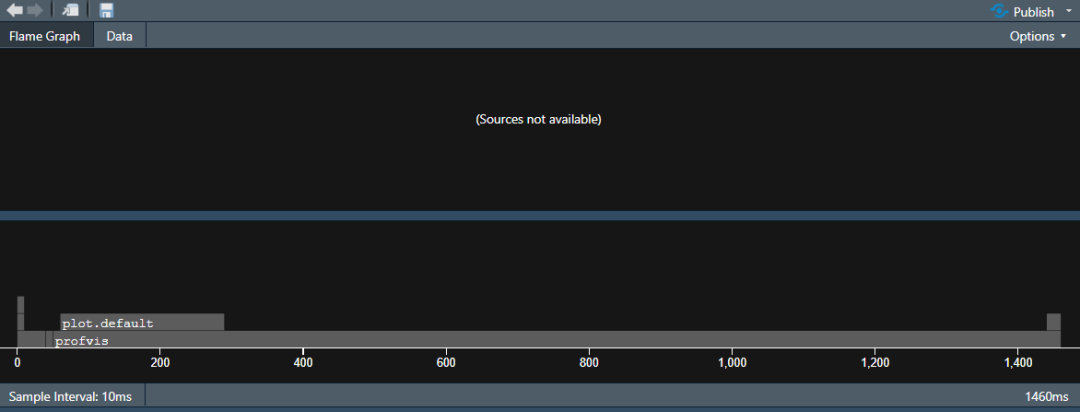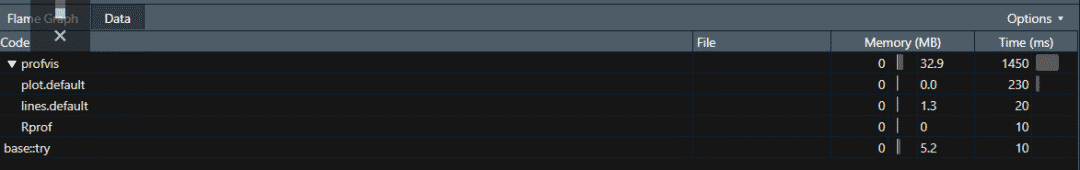# 开始profvis

``install.packages(c("profvis", "ggplot2movies")library(profvis)profvis({  data(movies,package = "ggplot2movies") # 加载数据  movies <- movies[movies\$Comedy ==1,]  plot(movies\$year, movies\$rating)  model <- loess(rating ~ year, data = movies) # 回归  j <- order(movies\$year)  lines(movies\$year[j], model\$fitted[j]) # 图中增加线})``结果显然是回归共了最多的时间，也是可以理解的。

# 高效的基础R

## if与ifelse函数

``marks <- runif(n=10e6, min=30, max=99)system.time({  result1 <- ifelse(marks >= 40, "pass", "fail")})# 用户 系统 流逝 2.64 0.06 2.70system.time({  for (mark in marks) {    if(mark >= 40) {result2 <-"pass"}else{result2 <- "fail"}  }})# 用户 系统 流逝 0.61 0.00 0.61``

## 逆向排序

decreasing = TRUE，比rev(sort(x)）快10%。

## 哪个索引是TRUE

which()

### 逻辑AND与OR

&和|是向量化的，非向量版本的&&和||，只在必要情况下执行第二个条件，注意不要使用它们操作向量。

### 行和列操作

apply()家族，rowSums()和colSums()。

# 并行计算

``library(parallel)detectCores()# 8``

## apply函数的并行版本

parapply() 等，多了一个cl函数指定CPU个数。是建立一个集群的意思，用完要停止，防止内存泄漏。

``cl <- makeCluster(8)...on.exit(stopCluster(cl)) # 如出错也退出，另一个常见用法，配合par()使用``

# Rcpp

C++是一个现代、快速并具有较强支持度的语言，包含各种库。Rcpp提供了一个友好的API，编写高性能代码，C++中瓶颈的典型是地址循环与递归函数。cppFunction()可以转换成R代码。

``add_r <- function(x, y) x * y # R语言版 # C++版library(Rcpp)cppFunction(double add_cpp(double x, double y){  double value = x * y;  return value})z <- microbenchmark(add_r(199999, 888888), add_cpp(199999, 888888))``